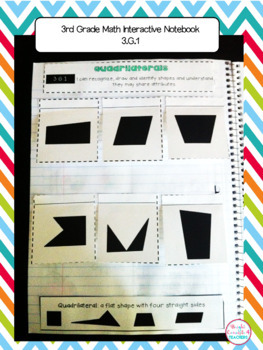# 3rd Grade Math Interactive Notebook {Common Core Aligned}3rd, Homeschool
Subjects
Standards
Resource Type
Formats Included
• PDF
Pages
120 pages

#### Also included in

1. Save with this bundle of THIRD Grade ELA & MATH Interactive Notebooks! This bundle includes the following 3 notebooks from my store:Math Interactive NotebookLanguage Interactive NotebookReading Interactive Notebook**If you purchase each notebook separately, it would cost \$38.00. You are getting
\$32.00
\$38.00
Save \$6.00

### Description

Interactive notebooks are a great learning tool in the classroom. They serve as a resource for reviewing standards, a reference tool, or even as an assessment.

This notebook covers all the 3rd Grade Common Core MATH standards (including Operations & Algebraic Thinking, Numbers and Operations in Base 10, Geometry, Measurement and Data, and Number and Operations-Fractions )

This product includes:

-Notebook cover

-Divider tabs

-Strand Dividers

-Pictures of EVERY page so you can have an example of how each activity can be used

-Student friendly "I can" statements at the top of each page.

I know your students will love making these notebooks as a method to reinforce all the CCSS math standards they have been taught.

Skills covered:

Operations and Algebraic Thinking:

-Understanding Multiplication with pictures

-Quotients

-Problem Solving with Multiplication and Missing Factors

-Problem Solving with Division

-Problem Solving with Division and Three Digit Numbers

-Multiplication and Division with an unknown number

-Properties of Multiplication

-Fact Families with Multiplication and Division

-Understanding Division

-Multiplication Chart

-Division Chart

-Multi-step Word Problems

-Using Variable Equations to solve word problems

-Rounding

-Estimation to solve word problems

-Addition, Subtraction, Multiplication and Division pattern tables

Numbers and Operations in Base 10:

-Place Value with Rounding

-Word Problem

-Multiplying one digit numbers by multiples of 10

Geometry:

-Solid Figures

-Plane Shapes

-Equal Parts

-Fractions

Measurement and Data:

-Telling Time

-Elapse Time

-Time Word Problems

-Time Lines

-Metric Measurement and Word Problem

-Bar Graphs

-Pictographs

-Measuring and Line Plots

-Area and Square Units

-Area of a rectangle

-Area word problems

-Perimeter

-Area v. Perimeter word problem

Number and Operations-Fractions

-Identifying Fractions

-Fractions on a Number line

-Equivalent Fractions

-Reducing Fractions

-Ordering Fractions

-Whole Numbers and Fractions

-Mixed Numbers

-Comparing Fractions

-Word Problems

3rd Grade Interactive Notebook BUNDLE {ELA & MATH}

3rd Grade ELA Interactive Notebook BUNDLE

More Math Interactive Notebooks:

Kindergarten Math Interactive Notebook

***************************************************

If you would like to get updates on NEW & CURRENT resources...

**************************************************

Email me at brightconcepts4teachers@gmail.com if you have any questions!

Total Pages
120 pages
N/A
Teaching Duration
1 Year
Report this Resource to TpT
Reported resources will be reviewed by our team. Report this resource to let us know if this resource violates TpT’s content guidelines.

### Standards

to see state-specific standards (only available in the US).
Interpret products of whole numbers, e.g., interpret 5 × 7 as the total number of objects in 5 groups of 7 objects each. For example, describe a context in which a total number of objects can be expressed as 5 × 7.
Interpret whole-number quotients of whole numbers, e.g., interpret 56 ÷ 8 as the number of objects in each share when 56 objects are partitioned equally into 8 shares, or as a number of shares when 56 objects are partitioned into equal shares of 8 objects each. For example, describe a context in which a number of shares or a number of groups can be expressed as 56 ÷ 8.
Use multiplication and division within 100 to solve word problems in situations involving equal groups, arrays, and measurement quantities, e.g., by using drawings and equations with a symbol for the unknown number to represent the problem.
Determine the unknown whole number in a multiplication or division equation relating three whole numbers. For example, determine the unknown number that makes the equation true in each of the equations 8 × ? = 48, 5 = __ ÷ 3, 6 × 6 = ?.
Apply properties of operations as strategies to multiply and divide. Examples: If 6 × 4 = 24 is known, then 4 × 6 = 24 is also known. (Commutative property of multiplication.) 3 × 5 × 2 can be found by 3 × 5 = 15, then 15 × 2 = 30, or by 5 × 2 = 10, then 3 × 10 = 30. (Associative property of multiplication.) Knowing that 8 × 5 = 40 and 8 × 2 = 16, one can find 8 × 7 as 8 × (5 + 2) = (8 × 5) + (8 × 2) = 40 + 16 = 56. (Distributive property.)